×#### Thank you for registering.

One of our academic counsellors will contact you within 1 working day.

Click to Chat

1800-1023-196

+91-120-4616500

CART 0

• 0

MY CART (5)

Use Coupon: CART20 and get 20% off on all online Study Material

ITEM
DETAILS
MRP
DISCOUNT
FINAL PRICE
Total Price: Rs.

There are no items in this cart.
Continue Shopping```Discriminant of a Quadratic EquationQuantity inside the square root (i.e. b2 – 4ac) discriminates the nature of root and so it is called discriminant (D) of the quadratic equation i.e.
D = b2 – 4ac. If you know the value of D of a given quadratic equation, you can be certain about the nature of its roots.

If D < 0                             roots are complex conjugates.

If D > 0                             roots are equal & real

If a, b, c Î Q (set of rational roots are real, different and rational number) and D is perfect square

If a, b, c Î Q (set of rational roots are real, different and rational number) and D is perfect irrational square

We have    f(x) = ax2 + bx + c where a, b, c Î R.

Now  f(x) = a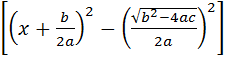= a= a[(x – a)(x – b)]

Where       a =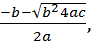b =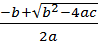……… (1)

These are the roots of the equation ax2 + bx + c = 0.

Now four possibilities arise :

Case I:

a, b, c are real numbers.

(i)     if a > 0, then D < 0 i.e. b2 – 4ac < 0

Þis purely imaginary

Hence from (1) both the roots a an b have to be imaginary in nature. (for conjugacy see next enquiry)

(ii)    When D = 0 i.e. b2 – 4ac = 0,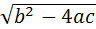= 0

\      from (i) a =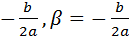Here both the roots a and b are real and equal.

Case II:

a, b, c are rational numbers.

(i)     If D = b2 – 4ac is a perfect square numbers.

Then the above said roots will reduce toHence, the roots a and b will be real and distinct.

(ii)    If D > 0 i.e. b2 – 4ac > 0 Þwould be a real number say (K). Thenwill be a rational numbers. Assume that if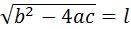.

then a =Here both the roots of the equation would be rational in nature.

In this way, we can know the nature of the roots of the given equation i.e. any type of quadratic equation can be solved.

Complex roots of a quadratic equation always occur in pair

If a quadratic equation has one complex root then the other must be complex conjugate of the first root.

Let a + ib is one root of the quadratic equation ax2 + bx + c = 0

so a(a + ib)2 + b(a + ib) + c = 0

or     a(a2 + 2abi – b2) + ba + ibb + c = 0

or     (aa2 + ab2 + bb + c) + (2aab + bb)i = 0

Equating real and imaginary parts,

aa2 – ab2 + ba + c) (2aab + bb)i = 0

or     (aa2 – 2aabi – ab2) + ba + bbi + c = 0

or     a(a – ib)2 + b(a – b) + c = 0

i.e. (a – ib) is also a root of the equation ax2 + bx + c = 0 and we know that every quadratic equation can have two and only two solution. So (a – ib) is the other solution of the equation which is complex conjugate of the first root (a + ib).

Basic Results

q  The quantity D(D=b2 – 4ac) is known as the discriminant of the quadratic equation.

q  The quadratic equation has real and equal roots if and only if D = 0 i.e. b2 – 4ac = 0.

q  The quadratic equation has real and distinct roots if and only if D > 0 i.e. b2 – 4ac . 0.

q  The quadratic equation has complex roots with non-zero imaginary parts if and only if D < 0 i.e. b2 – 4ac < 0.

q  If p + iq (p and q being real) is a root of the quadratic equation where i =, then p – iq is also a root of the quadratic equation.

q  If p +is an irrational root of the quadratic equation, then p –is also root of the quadratic equation provided that all the coefficients are rational.

q  The quadratic equation has rational roots if D is a perfect square and a, b, c are rational. If a = 1 and b, c are integers and the roots of the quadratic equation are rational, then the roots must be integers.

q  If the quadratic equation is satisfied by more than two numbers (real or complex), then it becomes an identity i.e. a = b = c = 0.

q  Let a and b be two roots of the given quadratic equation. Then a + b = –b/a and ab = c/a.

A quadratic equation, whose roots are a and b can be written as (x – a)
(x – b) = 0 i.e., ax2 + bx + c º a(x – a)(x – b).

Illustration:

Prove that the roots of the quadratic equation ax2 – 3bx – 4a = 0 are         real and distinct for all real and a and b.

Solution:

D = (–3b)2 –4(–4a) (a) = 9b2 + 16a2 which is always positive.

Hence the roots will be real and distinct.

Illustration:

Find the value of m for which the equation (1 + m)x2 –2(1 + 3m)x + (1 + 8m) = 0 has equal roots.

Solution:

Given equation is (1 + m)x2 –2(1 + 3m)x + (1 + 8m) = 0.

If roots are equal, then discriminant = 4(1+3m)2 –4(1+m)(1+8m) = 0

Þ m2 – 3m = 0 or m = 0, 3

Illustration:

If a and b be roots of equation ax2 + bx + c = 0, prove that a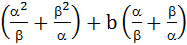= b.

Solution:

Here a + b = –b/a and ab = c/a.

Now, a==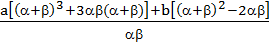=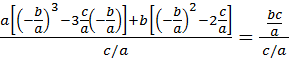= b0.

Enquiry:    Is it possible that a quadratic equation has more than two roots?

Let if possible, a, b, g be the three distinct roots of equation ax2 + bc + c = 0,

so     aa2 + ba + c = 0                                 …… (i)

and   ab2 + bb + c = 0                                 …… (ii)

and   ag2 + bg + c = 0                                  …… (iii)

from Eqn. (i) and (ii), we have,

a(a2 + b2) + b(a – b) = 0

Þ     a(a – b) (a + b) + b(a – b) = 0

Þ     a(a – b) [a(a + b) + b] = 0

since a ¹ 0 and a¹b,

so     a(a + b) + b = 0                                 …… (iv)

similarly from equation (ii) and (iii) we can have

a(b + g) + b = 0,                                 …… (v)

Now, eqn. (iv) and (v)

a(a – g) = 0

since a ¹ 0 so a = g

Hence, at the most two roots are possible.

Illustration:

Find the value of p if the equations 3x2 – 2x + p = 0 and 6x2 – 17x + 12 = 0 have a common root.

Solution:

Let a be the common root, then 3a2 – 2a + p = 0,           …… (1)

and 6a2 – 17a + 12 = 0                                                …… (2)

ÞÞa2 =and a =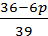Þ=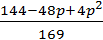Þ 312 – 221p = 432 – 144p + 12p2

Þ 12p2 + 77p + 120 = 0 Þ (4p + 15)(3p + 8) = 0 Þ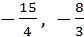.

To read more, Buy study materials of Quadratic Equation comprising study notes, revision notes, video lectures, previous year solved questions etc. Also browse for more study materials on Mathematics here.
```### Course Features

• 731 Video Lectures
• Revision Notes
• Previous Year Papers
• Mind Map
• Study Planner
• NCERT Solutions
• Discussion Forum
• Test paper with Video Solution